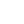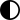There are many ways to convert a string into a number using JavaScript. But what does that look like in code?

In this article, I will show you 11 ways to convert a string into a number.

## How to convert a string to a number in JavaScript using the `Number()` function

One way to convert a string into a number would be to use the `Number()` function.

In this example, we have a string called `quantity` with the value of `"12"`.

``const quantity = "12";``

If we used the `typeof` operator on `quantity`, then it will return the type of string.

``````console.log(typeof quantity);
/* return "string" */``````

We can convert `quantity` into a number using the `Number` function like this:

``Number(quantity)``

We can check that it is now a number by using the `typeof` operator again.

``````console.log(typeof Number(quantity));
/* return "number" */``````

If you tried to pass in a value that cannot be converted into a number, then the return value would be `NaN` (Not a Number).

``````console.log(Number("awesome"));
/* return NaN */
``````

## How to convert a string to a number in JavaScript using the `parseInt()` function

Another way to convert a string into a number is to use the `parseInt()` function. This function takes in a string and an optional radix.

A radix is a number between 2 and 36 which represents the base in a numeral system. For example, a radix of 2 would represent the binary system, while a radix of 10 represents the decimal system.

We can use the `quantity` variable from earlier to convert that string into a number.

``````const quantity = "12";

console.log(parseInt(quantity, 10));``````

What happens if I try to change the `quantity` variable to `"12.99"`? Will the result using `parseInt()` be the number 12.99?

``````const quantity = "12.99";

console.log(parseInt(quantity, 10));
/* return 12 */``````

As you can see, the result is a rounded integer. If you wanted to return a floating point number, then you would need to use `parseFloat()`.

## How to convert a string to a number in JavaScript using the `parseFloat()` function

The `parseFloat()` function will take in a value and return a floating point number. Examples of floating point numbers would be 12.99 or 3.14.

If we modify our example from earlier to use `parseFloat()`, then the result would be the floating point number of 12.99.

``````const quantity = "12.99";

console.log(parseFloat(quantity));
/* return 12.99 */``````

If you have leading or trailing whitespace in your string, then `parseFloat()` will still convert that string into a floating point number.

``````const quantity = "   12.99    ";

console.log(parseFloat(quantity));
/* return 12.99 */``````

If the first character in your string cannot be converted into number, then `parseFloat()` will return `NaN`.

``````const quantity = "F12.99";

console.log(parseFloat(quantity));
/* return NaN */``````

## How to convert a string to a number in JavaScript using the unary plus operator (`+`)

The unary plus operator (`+`) will convert a string into a number. The operator will go before the operand.

``````const quantity = "12";

console.log(+quantity);
/* return 12 */``````

We can also use the unary plus operator (`+`) to convert a string into a floating point number.

``````const quantity = "12.99";

console.log(+quantity);
/* return 12.99 */``````

If the string value cannot be converted into a number then the result will be `NaN`.

``````const quantity = "awesome";

console.log(+quantity);
/* return NaN */``````

## How to convert a string to a number in JavaScript by multiplying the string by the number 1

Another way to convert a string into a number is to use a basic math operation. You can multiply the string value by 1 and it will return a number.

``````const quantity = "12";

console.log(quantity * 1);
/* return 12 */``````

As you can see, when we multiply the `quantity` value by 1, then it returns the number 12. But how does this work?

In this example, JavaScript is converting our string value to a number and then performing that mathematical operation.  If the string cannot be converted to a number, then the mathematical operation will not work and it will return `NaN`.

``````const quantity = "awesome";

console.log(quantity * 1);
/* return NaN */``````

This method will also work for floating point numbers.

``````const quantity = "10.5";

console.log(quantity * 1);
/* return 10.5 */``````

## How to convert a string to a number in JavaScript by dividing the string by the number 1

Instead of multiplying by 1, you can also divide the string by 1. JavaScript is converting our string value to a number and then performing that mathematical operation.

``````const quantity = "10.5";

console.log(quantity / 1);
/* return 10.5 */``````

## How to convert a string to a number in JavaScript by subtracting the number 0 from the string

Another method would be to subtract 0 from the string. Like before, JavaScript is converting our string value to a number and then performing that mathematical operation.

``````const quantity = "19";

console.log(quantity - 0);
/* return 19 */``````

## How to convert a string to a number in JavaScript using the bitwise NOT operator (`~`)

The bitwise NOT operator (`~`) will invert the bits of an operand and convert that value to a 32-bit signed integer. A 32-bit signed integer is a value that represents an integer in 32 bits (or 4 bytes).

If we use one bitwise NOT operator (`~`)  on a number, then it will perform this operation: -(x + 1)

``````console.log(~19);
/* return -20 */``````

But if we use two bitwise NOT operators (`~`), then it will convert our string to a number.

``````const quantity = "19";

console.log(~~quantity);
/* return -19 */``````

This method will not work for floating point numbers because the result would be an integer.

``````const quantity = "19.99";

console.log(~~quantity);
/* return -19 */``````

If you tried to use this method on non-numeric characters, then the result would be zero.

``````const quantity = "awesome";

console.log(~~quantity);
/* return 0 */``````

This method does have its limitations because it will start to break for values that are considered too large. It is important to make sure that your number is between the values of a signed 32 bit integer.

``````const quantity = "2700000000";

console.log(~~quantity);
/* return -1594967296 */``````

To learn more about the bitwise NOT operator (`~`) , please read up in the documentation.

## How to convert a string to a number in JavaScript using the `Math.floor()` function

Another way to convert a string to a number is to use the `Math.floor()` function. This function will round the number down to the nearest integer.

``````const quantity = "13.4";

console.log(Math.floor(quantity));
/* return 13 */``````

Just like in earlier examples, if we tried to use non-numeric characters, then the result would be `NaN`.

``````const quantity = "awesome";

console.log(Math.floor(quantity));
/* return NaN */``````

## How to convert a string to a number in JavaScript using the `Math.ceil()` function

The `Math.ceil()` function will round a number up to the nearest integer.

``````const quantity = "7.18";

console.log(Math.ceil(quantity));
/* return 8 */``````

## How to convert a string to a number in JavaScript using the `Math.round()` function

The `Math.round()` function will round the number to the nearest integer.

If I have the value of 6.3, then `Math.round()` will return 6.

``````const quantity = "6.3";

console.log(Math.round(quantity));
/* return 6 */``````

But if I changed that value to 6.5, then `Math.round()` will return 7.

``````const quantity = "6.5";

console.log(Math.round(quantity));
/* return 7 */``````

## Conclusion

In this article, I showed you 11 ways to convert a string to a number using JavaScript.

Here are the 11 different methods discussed in the article.

1. using the `Number()` function
2. using the `parseInt()` function
3. using the `parseFloat()` function
4. using the unary plus operator (`+`)
5. multiplying the string by the number 1
6. dividing the string by the number 1
7. subtracting the number 0 from the string
8. using the bitwise NOT operator (`~`)
9. using the `Math.floor()` function
10. using the `Math.ceil()` function
11. using the `Math.round()` function

Jessica Wilkins

Rating 5 of 5
Rate the article

## Comments for How to Convert a String to a Number in JavaScript

.com

Be the first to comment

Ние се грижим за вашите данни и ще използваме бисквитки само, за да подобрим вашето преживяване.
OK, със светла темаOK, с тъмна темаДекларация за поверителност
!
Сигурни ли сте, че искате да изчистите списъка с любими?